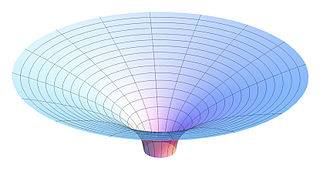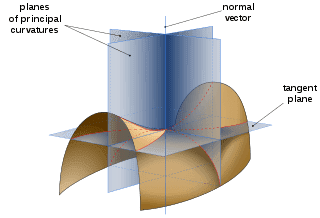# 2D curvature of Flamm's paraboloid

• A
Staff Emeritus
A question of sign. Is the curvature of Flamm's paraboloid positive or negative? If I've gotten the signs correct, it's a negative curvature. This is the opposite of the positive curvature of a sphere, and it implies that that geodesics drawn on Flamm's parabaloid should diverge. I think this makes sense, but a cross-check would be welcome.

•Dale

Negative curvature is correct.

•Dale
cianfa72
This is the opposite of the positive curvature of a sphere, and it implies that that geodesics drawn on Flamm's parabaloid should diverge.
If we consider it as a spacelike hypersurface of constant coordinate time t in Schwarzschild spacetime in Schwarzschild coordinate chart, I'm not sure it is actually a spacetime geodesic.

In other words: take two events (points) on the Flamm paraboloid. The spacetime spacelike curve joining them that is a geodesic in the induced metric on the Flamm paraboloid from the Schwarzschild spacetime metric, may not be a geodesic of the Schwarzschild spacetime itself.

A question of sign. Is the curvature of Flamm's paraboloid positive or negative? If I've gotten the signs correct, it's a negative curvature.
Intuitive approach: In the 3D embedding it's obviously more like a saddle than a sphere.If you try to construct tangent circles for the principal extrinsic curvatures in the embedding space, they will be on opposite sides, which implies negative intrinsic curvature.a geodesic in the induced metric on the Flamm paraboloid from the Schwarzschild spacetime metric, may not be a geodesic of the Schwarzschild spacetime itself.
You can't really tell, because a spatial Flamm geodesic doesn't uniquely define a worldline in spacetime. But if you go the other way and project a geodesic in spacetime onto the spatial Flamm paraboloid. then it's not a spatial Flamm geodesic in general.

For example: Two nearby radially free falling bodies converge in space, so the spatial projections of their geodesic worldlines cannot be geodesics on the negatively curved Flamm paraboloid

•italicus
italicus
In other words: take two events (points) on the Flamm paraboloid. The spacetime spacelike curve joining them that is a geodesic in the induced metric on the Flamm paraboloid from the Schwarzschild spacetime metric, may not be a geodesic of the Schwarzschild spacetime itself.
Are you sure that the curve joining the events is a spacelike curve?
This paraboloid reminds me the Beltrami pseudosphere of differential 3D geometry, which curvature is negative.

Last edited:
cianfa72
Are you sure that the curve joining the events is a spacelike curve?
Why not ? In the exterior of Schwarzschild spacetime you get a Flamm paraboloid as a hypersurface of constant coordinate time ##t## (##t## is the Schwarzschild chart's coordinate time). At each point on such hypersurface (the 2D Flamm paraboloid when we drop a spatial dimension) the directions having ##dt=0, d \theta=0## are all spacelike (they 'live' in the vector subspace of the spacetime tangent vector space that is tangent to the hypersurface submanifold).

Hence each curve 'drawn' on the Flamm paraboloid is actually a spacelike curve of the 'underlying' exterior Schwarzschild spacetime.

Last edited:
Staff Emeritus
I believe that a geodesic on the Flamm paraboloid would represent a spatial geodesic in the t=0, ##\theta=0## plane of the Schwarzschild metric, because the line element of the Flamm paraboloid expressed in r , ##\phi## coordiantes is the same line element as the Schwarzshild line element with dt=##d\theta##=0.

•cianfa72
Staff Emeritus
If one looks at null geodesics (the geodesics of light) in the Schwarzschild space-time, I believe one will find that there is more deflection of the former than the later, so they definitely won't be the same.

Consider a light beam moving in the ##\hat{\theta}## direction at the point of closest approach to the central mass in an orthonormal basis of the Schwarzschild metric with coordinates (t,r,##\theta##,##\phi##). I've followed MTW's notation by using 'hats' to indicate the basis vectors of the orthonormal basis, to distinguish them from the coordinate basis.

Compare it to a purely spatial geodesic "moving" in the ##\hat{\theta}## direction. We have some affine parameter s for both the null geodesic and the spatial geodesic, the null geodesic has components ##dt/ds## and ##d\theta/ds##, while the spatial geodesic only has a component ##d\theta/ds##.

Both geodesics will be deflected in the ##\hat{r}## direction, but the magnitude of the deflection will differ. As mentioned previously using slightly different notation, the spatial geodesic will have a 4-velocity in the orthonormal basis of (0,0,1,0), while the light beam will have a 4-velocity of (1,0,1,0).

We will assign the indices 0,1,2,3 to the t,r,theta,phi coordinates, respectively. The nonzero 4-velocity components are all unity, and we will assume the separation vector is unity as well, to make the math easier.

Then the geodesic deviation of the spatial geodesic will have one nonzero component, ##R^{\hat{1}}{}_{\hat{2}\hat{1}\hat{2}}##, while the geodesic deviation of the light will have two nonzero components, ##R^{\hat{1}}{}_{\hat{0}\hat{1}\hat{0}} + R^{\hat{1}}{}_{\hat{2}\hat{1}\hat{2}}##

We see the light has a higher geodesic deviation. I actually expected the light deflection to be double, but that's not quite what I'm getting at the moment, I'm not positive whether my calculation or my expectation was wrong. But one can definitely see the geodesic deviation between nearby null geodesics is greater than the geodesic deviation between nearby spatial geodesics, so they aren't the same. In words, "gravity" and "spatial curvature" both deflect the light, while only "spatial curvature" deflects the spatial geodesic.

One can also see that the light beams do diverge, as do the null geodesics, consistent with the negative curvature. But they diverge at different rates.